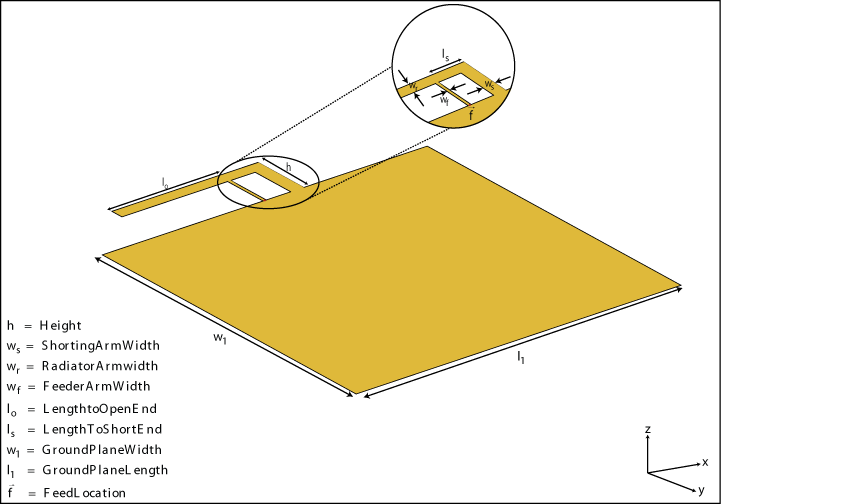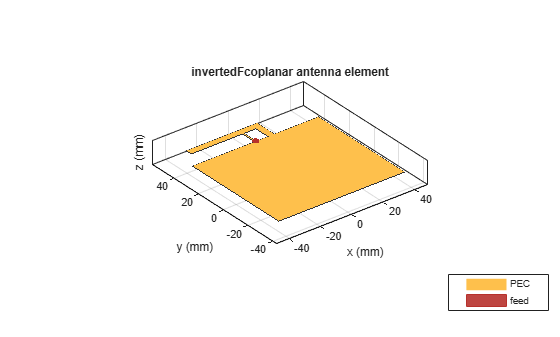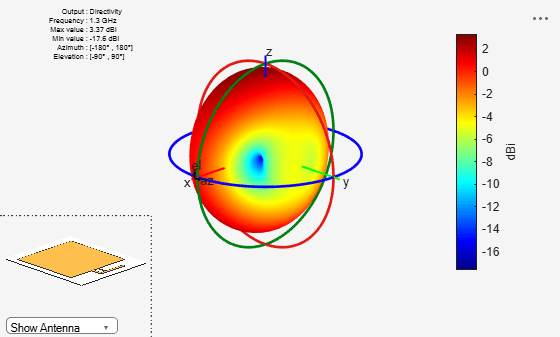# invertedFcoplanar

Create inverted-F antenna in same plane as rectangular ground plane

## Description

The `invertedFcoplanar` object is a coplanar inverted-F antenna with a rectangular ground plane. By default, the dimensions are chosen for an operating frequency of 1.7 GHz. Coplanar inverted-F antennas are used in RFID tags and Internet of Things (IoT) applications. This antenna is an altered version of the inverted-F antenna, providing a low-profile antenna with more design parameters and a wider bandwidth.## Creation

### Syntax

``fco = invertedFcoplanar``
``fco = invertedFcoplanar(Name,Value)``

### Description

example

``` `fco = invertedFcoplanar` creates a coplanar inverted-F antenna with the rectangular ground plane. By default, the antenna dimensions are for an operating frequency of 1.7 GHz.```

example

``` `fco = invertedFcoplanar(Name,Value)` creates a coplanar inverted-F antenna, with additional properties specified by one or more name-value pair arguments. `Name` is the property name and `Value` is the corresponding value. You can specify several name-value pair arguments in any order as `Name1`, `Value1`, `...`, `NameN`, `ValueN`. Properties not specified retain their default values.```

## Properties

expand all

Width of radiating arm, specified as the comma-separated pair consisting of `'RadiatorArmWidth'`and a scalar in meters.

Example: `'RadiatorArmWidth',0.05`

Data Types: `double`

Width of feeding arm, specified as a scalar in meters.

Example: `'FeederArmWidth',0.05`

Data Types: `double`

Width of shorting arm, specified as a scalar in meters.

Example: `'ShortingArmWidth',1`

Data Types: `double`

Height of antenna from ground plane, specified as a scalar in meters.

Example: `'Height',0.0800`

Data Types: `double`

Length of the stub from feed to the open-end, specified as a scalar in meters.

Example: `'LengthToOpenEnd',0.050`

Data Types: `double`

Length of the stub from feed to the shorting end, specified as a scalar in meters.

Example: `'LengthToShortEnd',0.035`

Data Types: `double`

Length of the ground plane, specified as a scalar in meters.

Example: `'GroundPlaneLength',0.035`

Data Types: `double`

Width of the ground plane, specified as a scalar in meters.

Example: `'GroundPlaneWidth',0.035`

Data Types: `double`

Signed distance from center of ground plane, specified as a scalar in meters.

Example: `'FeedOffset',0.06`

Data Types: `double`

Type of the metal used as a conductor, specified as a metal material object. You can choose any metal from the `MetalCatalog` or specify a metal of your choice. For more information, see `metal`. For more information on metal conductor meshing, see Meshing.

Example: ```m = metal('Copper'); 'Conductor',m```

Example: ```m = metal('Copper'); ant.Conductor = m```

Lumped elements added to the antenna feed, specified as a lumped element object. For more information, see `lumpedElement`.

Example: `'Load',lumpedelement`. `lumpedelement` is the object for the load created using `lumpedElement`.

Example: ```fco.Load = lumpedElement('Impedance',75)```

Tilt angle of the antenna, specified as a scalar or vector with each element unit in degrees. For more information, see Rotate Antennas and Arrays.

Example: `Tilt=90`

Example: `Tilt=[90 90]`,`TiltAxis=[0 1 0;0 1 1]` tilts the antenna at 90 degrees about the two axes defined by the vectors.

Note

The `wireStack` antenna object only accepts the dot method to change its properties.

Data Types: `double`

Tilt axis of the antenna, specified as:

• Three-element vector of Cartesian coordinates in meters. In this case, each coordinate in the vector starts at the origin and lies along the specified points on the X-, Y-, and Z-axes.

• Two points in space, each specified as three-element vectors of Cartesian coordinates. In this case, the antenna rotates around the line joining the two points in space.

• A string input describing simple rotations around one of the principal axes, 'X', 'Y', or 'Z'.

Example: `TiltAxis=[0 1 0]`

Example: `TiltAxis=[0 0 0;0 1 0]`

Example: `TiltAxis = 'Z'`

Data Types: `double`

## Object Functions

 `show` Display antenna, array structures or shapes `info` Display information about antenna or array `axialRatio` Axial ratio of antenna `beamwidth` Beamwidth of antenna `charge` Charge distribution on antenna or array surface `current` Current distribution on antenna or array surface `design` Design prototype antenna or arrays for resonance around specified frequency `efficiency` Radiation efficiency of antenna `EHfields` Electric and magnetic fields of antennas; Embedded electric and magnetic fields of antenna element in arrays `impedance` Input impedance of antenna; scan impedance of array `mesh` Mesh properties of metal, dielectric antenna, or array structure `meshconfig` Change mesh mode of antenna structure `optimize` Optimize antenna or array using SADEA optimizer `pattern` Radiation pattern and phase of antenna or array; Embedded pattern of antenna element in array `patternAzimuth` Azimuth pattern of antenna or array `patternElevation` Elevation pattern of antenna or array `rcs` Calculate and plot radar cross section (RCS) of platform, antenna, or array `returnLoss` Return loss of antenna; scan return loss of array `sparameters` Calculate S-parameter for antenna and antenna array objects `show` Display antenna, array structures or shapes `vswr` Voltage standing wave ratio of antenna

## Examples

collapse all

Create a default coplanar inverted-F antenna and view it.

`fco = invertedFcoplanar`
```fco = invertedFcoplanar with properties: RadiatorArmWidth: 0.0040 FeederArmWidth: 1.0000e-03 ShortingArmWidth: 0.0040 LengthToOpenEnd: 0.0350 LengthToShortEnd: 0.0100 Height: 0.0100 GroundPlaneLength: 0.0800 GroundPlaneWidth: 0.0700 FeedOffset: 0 Conductor: [1x1 metal] Tilt: 0 TiltAxis: [1 0 0] Load: [1x1 lumpedElement] ```
`show(fco)`Create a coplanar inverted-F antenna of height 0.014 m, ground plane length 0.1 m, and ground plane width 0.1 m.

``` fco = invertedFcoplanar('Height',14e-3,'GroundPlaneLength', 100e-3, ... 'GroundPlaneWidth', 100e-3);```

Plot the radiation pattern of the above antenna.

` pattern(fco,1.30e9)`Balanis, C. A. Antenna Theory. Analysis and Design. 3rd Ed. Hoboken, NJ: John Wiley & Sons, 2005.

 Stutzman, W. L. and Gary A. Thiele. Antenna Theory and Design. 3rd Ed. River Street, NJ: John Wiley & Sons, 2013.